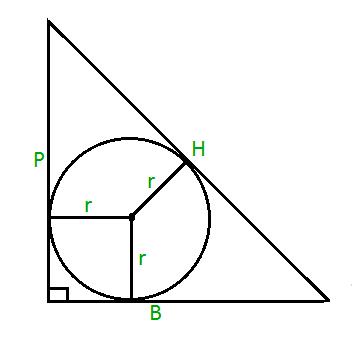# Area of Incircle of a Right Angled Triangle

Given the P, B and H are the perpendicular, base and hypotenuse respectively of a right angled triangle. The task is to find the area of the incircle of radius r as shown below:Examples:

Input: P = 3, B = 4, H = 5
Output: 3.14

Input: P = 5, B = 12, H = 13
Output: 12.56

## Recommended: Please try your approach on {IDE} first, before moving on to the solution.

Approach: Formula for calculating the inradius of a right angled triangle can be given as r = ( P + B – H ) / 2.
And we know that the area of a circle is PI * r2 where PI = 22 / 7 and r is the radius of the circle.
Hence the area of the incircle will be PI * ((P + B – H) / 2)2.

Below is the implementation of the above approach:

## C

 `// C program to find the area of ` `// incircle of right angled triangle ` `#include ` `#define PI 3.14159265 ` ` `  `// Function to find area of ` `// incircle ` `float` `area_inscribed(``float` `P, ``float` `B, ``float` `H) ` `{ ` `    ``return` `((P + B - H) * (P + B - H) * (PI / 4)); ` `} ` ` `  `// Driver code ` `int` `main() ` `{ ` `    ``float` `P = 3, B = 4, H = 5; ` `    ``printf``(``"%f"``, ` `           ``area_inscribed(P, B, H)); ` `    ``return` `0; ` `} `

## Java

 `// Java code to find the area of inscribed ` `// circle of right angled triangle ` `import` `java.lang.*; ` ` `  `class` `GFG { ` ` `  `    ``static` `double` `PI = ``3.14159265``; ` ` `  `    ``// Function to find the area of ` `    ``// inscribed circle ` `    ``public` `static` `double` `area_inscribed(``double` `P, ``double` `B, ``double` `H) ` `    ``{ ` `        ``return` `((P + B - H) * (P + B - H) * (PI / ``4``)); ` `    ``} ` ` `  `    ``// Driver code ` `    ``public` `static` `void` `main(String[] args) ` `    ``{ ` `        ``double` `P = ``3``, B = ``4``, H = ``5``; ` `        ``System.out.println(area_inscribed(P, B, H)); ` `    ``} ` `} `

## Python3

 `# Python3 code to find the area of inscribed  ` `# circle of right angled triangle ` `PI ``=` `3.14159265` `     `  `# Function to find the area of  ` `# inscribed circle ` `def` `area_inscribed(P, B, H): ` `    ``return` `((P ``+` `B ``-` `H)``*``(P ``+` `B ``-` `H)``*``(PI ``/` `4``)) ` `     `  `# Driver code ` `P ``=` `3` `B ``=` `4` `H ``=` `5` `print``(area_inscribed(P, B, H)) `

## C#

 `// C# code to find the area of ` `// inscribed circle ` `// of right angled triangle ` `using` `System; ` ` `  `class` `GFG { ` `    ``static` `double` `PI = 3.14159265; ` ` `  `    ``// Function to find the area of ` `    ``// inscribed circle ` `    ``public` `static` `double` `area_inscribed(``double` `P, ``double` `B, ``double` `H) ` `    ``{ ` `        ``return` `((P + B - H) * (P + B - H) * (PI / 4)); ` `    ``} ` ` `  `    ``// Driver code ` `    ``public` `static` `void` `Main() ` `    ``{ ` `        ``double` `P = 3.0, B = 4.0, H = 5.0; ` `        ``Console.Write(area_inscribed(P, B, H)); ` `    ``} ` `} `

## PHP

 ` `

Output:

```3.141593
```

Don’t stop now and take your learning to the next level. Learn all the important concepts of Data Structures and Algorithms with the help of the most trusted course: DSA Self Paced. Become industry ready at a student-friendly price.

My Personal Notes arrow_drop_upCheck out this Author's contributed articles.

If you like GeeksforGeeks and would like to contribute, you can also write an article using contribute.geeksforgeeks.org or mail your article to contribute@geeksforgeeks.org. See your article appearing on the GeeksforGeeks main page and help other Geeks.

Please Improve this article if you find anything incorrect by clicking on the "Improve Article" button below.

Article Tags :
Practice Tags :

Be the First to upvote.

Please write to us at contribute@geeksforgeeks.org to report any issue with the above content.